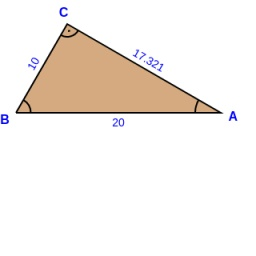# Coefficient 6672

In the triangle ABC is [AB] = 20cm, [BC] = 10cm, A = 30 °. Construct a triangle A'B'C' similar to triangle ABC if the similarity coefficient is 0.5

a1 =  5 cm
c1 =  10 cm
A1 =  30 °

### Step-by-step explanation:

Try calculation via our triangle calculator.Did you find an error or inaccuracy? Feel free to write us. Thank you!

Tips for related online calculators